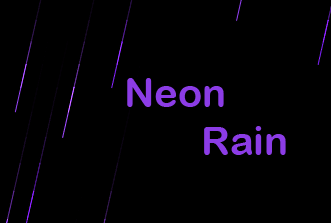/

# Amazing Neon Rain Using HTML5 Canvas API

Hi There, HTML5 Canvas is one of the most amazing features of HTML5. We can create flash like rich effect and games using HTML5 Canvas API. We can draw any kind of shape on HTML5 Canvas. When we combine our code with timers(`settimeout` or `requestAnimFrame`) we can get some nice movements on our objects . So that we can create an amazing game. As HTML5 Canvas API is a subset of JavaScript. We can use all JavaScript features like event handling, or string manipulation, etc…. If you want to learn more about HTML5 Canvas go thought these tutorials. In today’s tutorial we will create this amazing Neon rain effect using HTML5 Canvas API. Let’s start.demo

First, set an HTML page to display your canvas.

## index.html

``````<!doctype html>
<html lang="en">

<meta charset="UTF-8">
<title>Document</title>
<style>
* {
margin: 0;
border: 0;
}
body {
background: #000;
}
</style>

<body>
<script type="text/javascript" src="app.js"></script>
</body>

</html>
``````

This is a simple HTML without anybody. We just added app.js file at bottom.

## app.js

``````"use strict";
//crossbrowser requestAnimationFrame shim
window.requestAnimFrame = (function() {
return window.requestAnimationFrame ||
window.webkitRequestAnimationFrame ||
window.mozRequestAnimationFrame ||
function(callback) {
window.setTimeout(callback, 1000 / 60);
};
})();

var App = function() {
var base = this,
canvas, ctx, drops = [],
color = 120,
w = window.innerWidth,
h = window.innerHeight;
//App initilizer
base.init = function() {
base.setup();
base.loop();
};
//setting up html5 canvas
base.setup = function() {
canvas = document.createElement('canvas');
canvas.width = w;
canvas.height = h;
document.body.appendChild(canvas);
ctx = canvas.getContext('2d');
};
//to create random umber within the range.
base.rand = function(min, max) {
return Math.random() * (max - min) + min;
};
//to calculte distance between tow coords
base.calDistance = function(x1, y1, x2, y2) {
return Math.sqrt(Math.pow((x2 - x1), 2) + Math.pow((y2 - y1), 2));
};
//The rain object
base.Rain = function(ix, iy, ex, ey) {
var rain = this,
x = ix,
y = iy,
distanceTraveled = 0,
speed = 2,
acceleration = 1,
distanceToTarget = 0,
coords = [],
coordinateCount = 2;
//initilizing rain object
rain.init = function() {
angle = Math.atan2(ey - iy, ex - ix);
brightness = base.rand(50, 70);
distanceToTarget = base.calDistance(ix, iy, ex, ey);
// populate initial coordinate collection with the current coords
while (coordinateCount--) {
coords.push([ix, iy]);
}
};
//drawing rain drop
rain.draw = function() {
ctx.beginPath();
// move to the last tracked coordinate in the set, then draw a line to the current x and y
ctx.moveTo(coords[coords.length - 1], coords[coords.length - 1]);
ctx.lineTo(x, y);
ctx.strokeStyle = 'hsl(625, 100%, ' + brightness + '%)';
ctx.stroke();
ctx.beginPath();
};
//updating rain drop
rain.update = function(i) {
coords.pop();
coords.unshift([x, y]);
// speed up the rain drops
speed += acceleration;
// get the current velocities based on angle and speed
var vx = Math.cos(angle) * speed,
vy = Math.sin(angle) * speed;
distanceTraveled = base.calDistance(ix, iy, x + vx, y + vy);

if (distanceTraveled >= distanceToTarget) {
drops.splice(i, 1);
} else {
x += vx;
y += vy;
}
};
rain.init();
};
//loop animation
base.loop = function() {
requestAnimFrame(base.loop);
ctx.globalCompositeOperation = 'destination-out';
ctx.fillStyle = 'rgba(0, 0, 0, 0.5)';
ctx.fillRect(0, 0, w, h);
ctx.globalCompositeOperation = 'lighter';
// loop over each drops, draw it, update it
var i = drops.length;
while (i--) {
drops[i].draw();
drops[i].update(i);
}
var sx = base.rand(0, w + 200);
drops.push(new base.Rain(sx, 0, sx - 200, h, color));
};
base.events = function() {